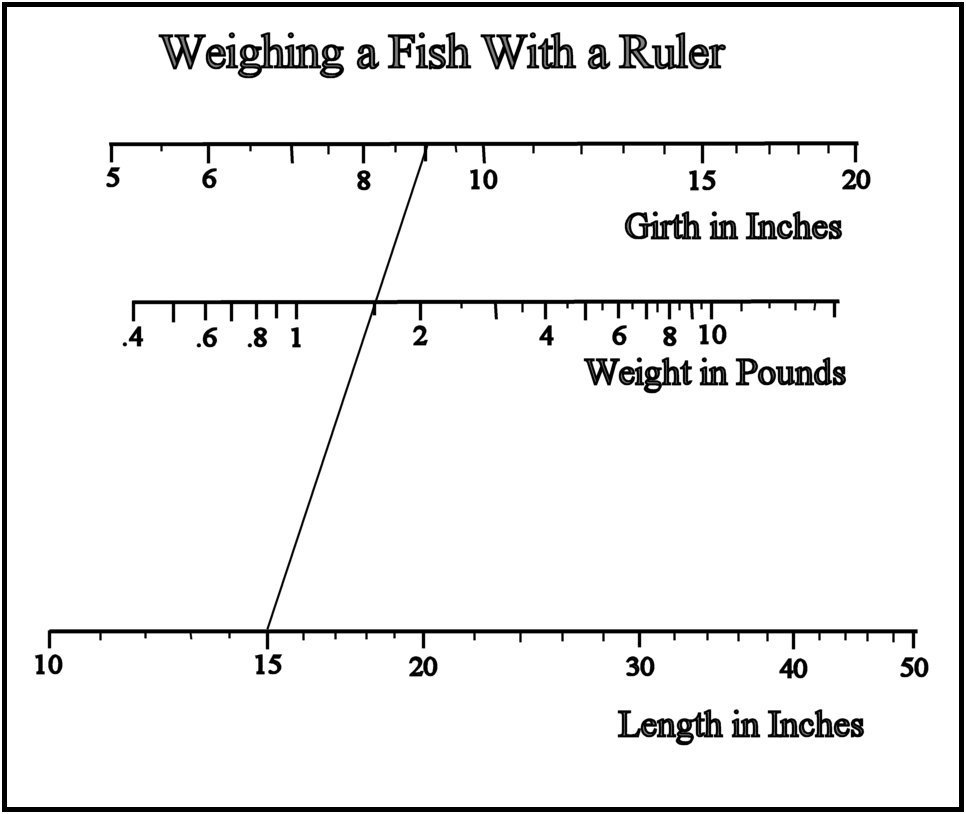How Much Does It Weigh?

There are times when we catch a nice sized fish and we wonder how much does it weigh? There is a formula to determine the weight of a trout if we know how the length and girth of the fish. This formula works for medium size fish with body plan of a trout. It is not accurate for small fish and very large fish.  The measurements are in inches and the weight will be in pounds.

The formula for the mathematical minded is as follows:   Weight = Girth X Girth X Length / 800.

The example shown is for a 15 inch fish with a 9 inch girth. Print out this diagram and take it fishing with you. No responsibility is assumed for accuracy.